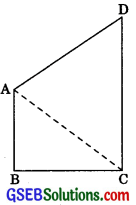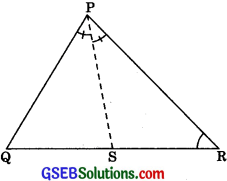# GSEB Solutions Class 9 Maths Chapter 7 Triangles Ex 7.4

Gujarat Board GSEB Solutions Class 9 Maths Chapter 7 Triangles Ex 7.4 Textbook Questions and Answers.

## Gujarat Board Textbook Solutions Class 9 Maths Chapter 7 Triangles Ex 7.4

Question 1.
Show that in a right-angled triangle, the hypotenuse is the longest side.
Solution:
Given: Right ΔABC which is right-angled at
B, i.e., ∠B 900,
Then ∠A + ∠B + ∠C = 180°
(by angle sum property)∠A + 90° + ∠C = 180°
∠A + ∠C = 90°
∴ AC > BC (Side opposite to greater angle is longer)
and ∠B>∠C
∴ AC > AB (Side opposite to greater angle is longer)
Hence, AC, the hypotenuse is the longest side.Question 2.
Infiguresides AB and AC of ΔABC are extended to points P and Q respectively. Also, ∠PBC < ∠QCB. Show that AC > AB.Solution:
∠PBC < ∠QCB (given)
⇒ 180°- ∠PBC>180°- ∠QCB
⇒∠ABC > ∠ACB
∴ AC > AB (Side opposite to greater angle is longer)

Question 3.
In the figure,∠B<∠A and ∠C<∠D. Show that AD∠BCSolution:
∠B <∠A (given)
∠A >∠B
OB > OA ……….(1)
(Side opposite to greater angle is longer)
Now ∠C <∠D (given)
∴ ∠D > ∠C
∴ OC > OD ……….(2)
(Side opposite to greater angle is longer)
Adding eqn. (1) and (2), we get
OB + OC > OA + ODQuestion 4.
AB and CD are respectively the smallest and longest sides of a quadrilateral ABCD (see figure). Show that ∠A> ∠C and ∠B> ∠D.Solution:
Construction: Join AC.
Proof: In ΔABC,
AB AB
∠BAC > ∠BCA …….. (1) (Angle opposite to longer side is greater)
In ΔACD
∴ ∠CMD > ∠ACD ……(2) (Angle
opposite to longer side is greater)
∠BAC + ∠CAD> ∠BCA + ∠ACD
⇒ ∠A > ∠C
Similarly, join B to D and we can prove
∠B > ∠D

Question 5.
In figure, PR > PQ and PS bisects ∠QPR. Prove that ∠PSR> ∠PSQ.Given:
In figure PR>PQ
and PS bisects ∠QPR.
To Prove:
∠PSR > ∠PSQ
Proof In ΔPQR,
PR > PQ (given)
∠PQR > ∠PRQ
∠PQS > ∠PRS ……..(1)
∠QPS = ∠RPS …….(2) (PS is the angle bisector of ∠QPR)
Adding ∠QPS on both sides in eqn. (1)
∠PQS + ∠QPS > ∠PRS + ∠QPS ……..(3)
From eqn. (2) and (3)
⇒ ∠PQS + ∠QPS> ∠PRS + ∠RPS …….(4)
⇒ ∠PSQ = ∠PRS + ∠RPS ……….(5) (By exterior angle theorem)
and ∠PSR = ∠QPS + ∠PQS …….(6) (by exterior angle theorem)
From eqn. (4), (5) and (6), we have
∠PSR > ∠PSQQuestion 6.
Show that of all line segments drawn from a given point not on it, the perpendicular line segment is the shortest.
Solution:
Given: A line I and P is a point not lying online l and PQ ⊥ l and R is any point on l other than Q.
To Prove:  PQ < PR
Proof: in Δ PQR
∠Q = 90º∠P + ∠Q + ∠R = 180° m(by angle sum property)
⇒ ∠P + 90° + ∠R = 180°
⇒ ∠P + ∠R = 90°
Hence ∠R is an acute angle.
∴ ∠Q > ∠R
(Side opposite to greater angle is longer)
∴ PR >PQ
⇒ PQ<PR
Hence perpendicular line segment is the shortest.
Hence the perpendicular line segment is the shortest.